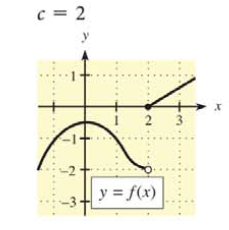Chapter 9.1, Problem 10E### Mathematical Applications for the ...

11th Edition
Ronald J. Harshbarger + 1 other
ISBN: 9781305108042

#### Solutions

Chapter
Section### Mathematical Applications for the ...

11th Edition
Ronald J. Harshbarger + 1 other
ISBN: 9781305108042
Textbook Problem

# In Problems 7-10, use the graph of y = f ( x ) and the given c-value to find the following, whenever they exist.(a) lim x → c − f ( x ) (b) lim x → c + f ( x ) (c) lim x → c f ( x ) (d) f ( c ) c = 2(a)

To determine

The value of limxcf(x) by the use of the graph,Explanation

Given Information:

The value of c is 2. The graph of y=f(x) is

Explanation:

Consider the provided graph,

The value of c is equal to 2.

The limit from the left is represented by limx2f(x), that is, the values of f(c) but c<2

(b)

To determine

The value of limxc+f(x) by the use of the graph,(c)

To determine

The value of limxcf(x) by the use of the graph,(d)

To determine

The value of f(c) by the use of the graph,### Still sussing out bartleby?

Check out a sample textbook solution.

See a sample solution

#### The Solution to Your Study Problems

Bartleby provides explanations to thousands of textbook problems written by our experts, many with advanced degrees!

Get Started

#### Divide: 9x53x3

Elementary Technical Mathematics

#### True or False: is a rational function.

Study Guide for Stewart's Single Variable Calculus: Early Transcendentals, 8th

#### True or False: If and , then .

Study Guide for Stewart's Multivariable Calculus, 8th